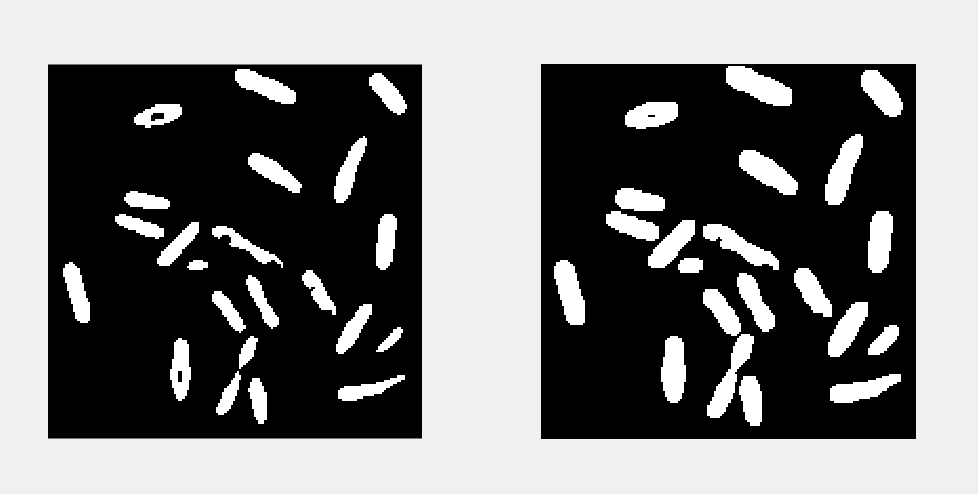# 形态学图像处理

Posted by jjx on December 28, 2016

• 二值图像的基本形态学运算， 包括腐蚀、膨胀、开和闭。
• 二值形态学的经典应用， 包括击中击不中变换、边界提取和跟踪、区域填充、提取连通分量、细化和像素化， 以及凸壳
• 灰度图像的形态学运算， 包括灰度腐蚀、灰度膨胀、灰度开和灰度闭
• 本章的典型案例分析
• 在人脸局部图像中定位嘴的中心
• 显微镜下图像的细菌计数
• 利用顶帽变换（top-hat）技术解决光照不均问题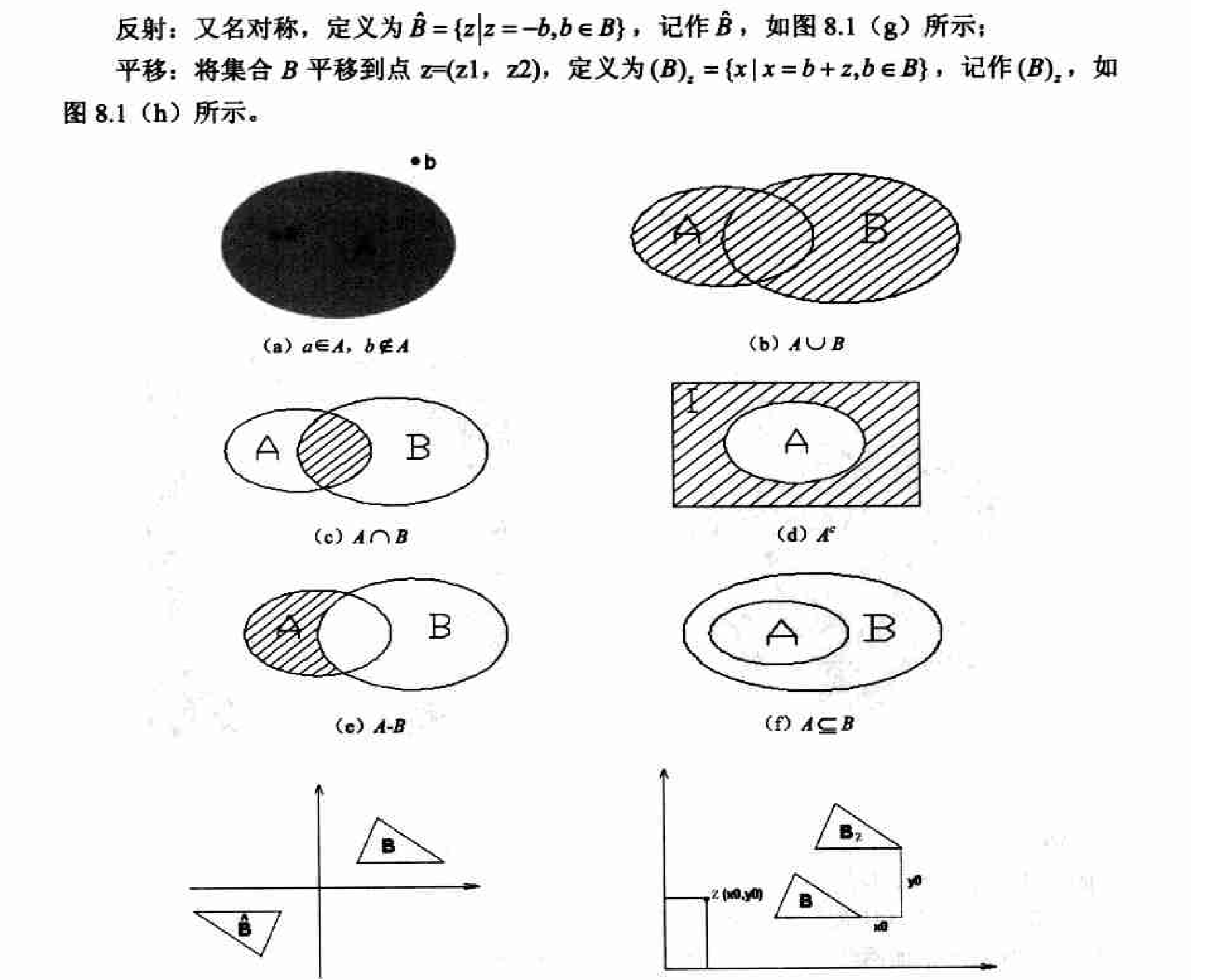### 二值图像中的基本形态学运算

#### 腐蚀及其实现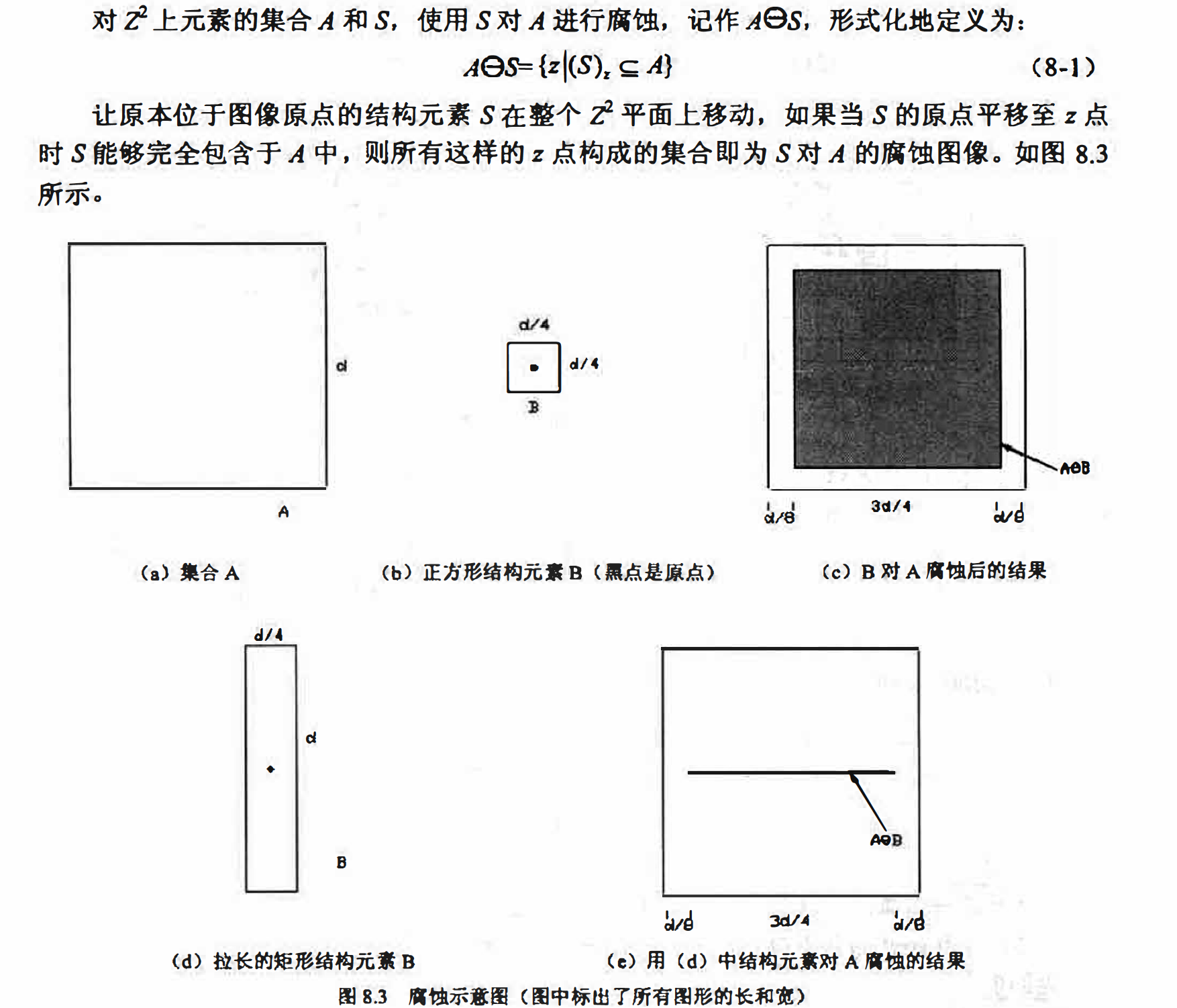matlab实现
Matlab中与腐蚀相关的两个常用函数为imerode和strel。
imerode函数用于完成图像腐蚀．其常用调用形式如下:I2 = imrode(I,SE)
I为原始图像，可以是二位或灰度图像（对应于灰度腐蚀）．
SE是由strel函数返回的自定义或预设的结构元素对象．

strel函数可以为各种常见形态学运算生成结构元素SE， 当生成供二值形态学使用的结构元素肘， 其调用形式为:SE=strl(shape,parameter)
shape指定了结构元素的形状， 其常用合法取值如在8.1所示．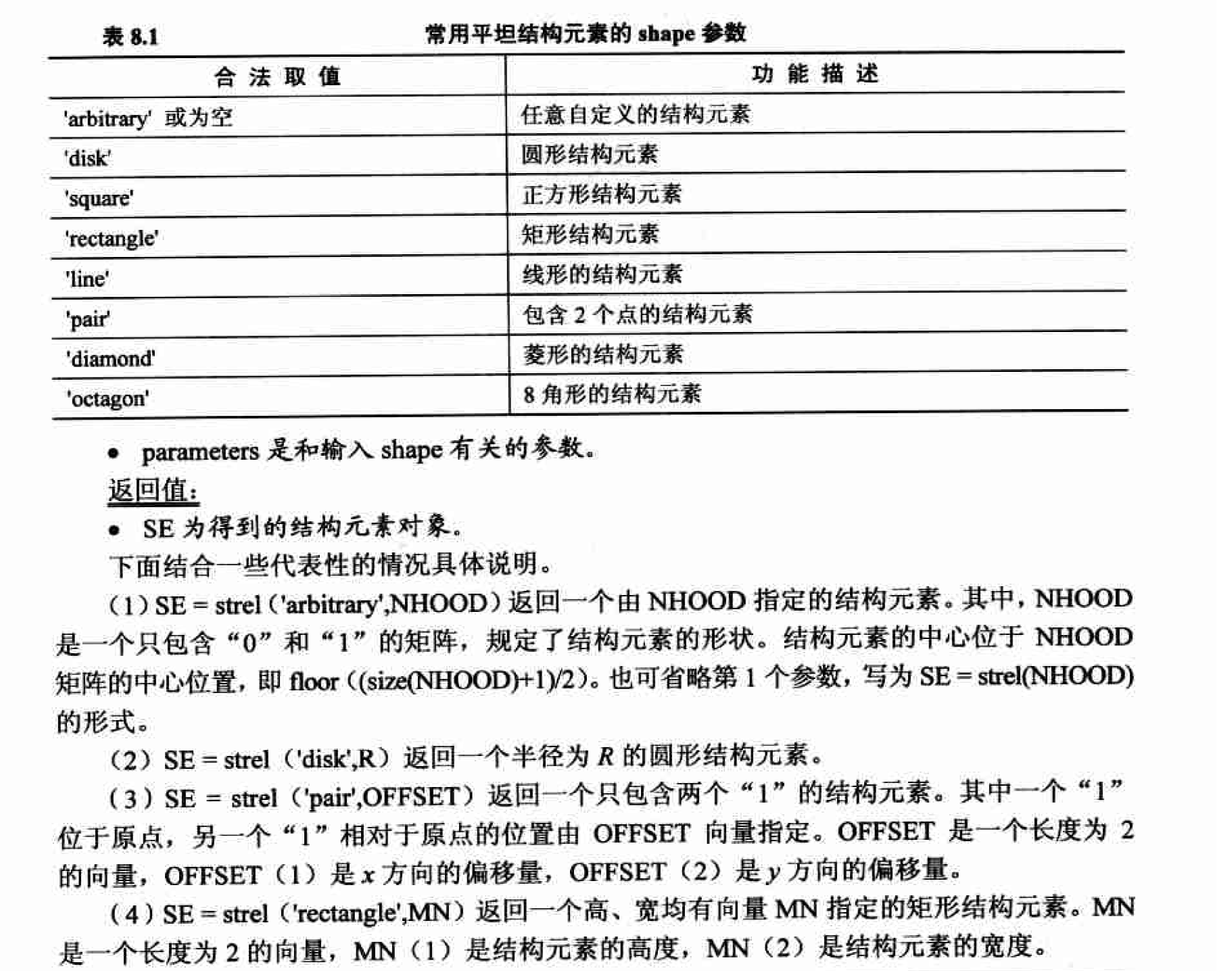I = imread('erode_dilate.bmp');
se = strel('square',3);
Ib = imerode(I,se);
se = strel([0 1 0;1 1 1;0 1 0]);
Ic = imerode(I,se);
se = strel('square',5);
Id = imerode(I,se);

figure;
subplot(2,2,1);
imshow(I);
subplot(2,2,2);
imshow(Ib);
subplot(2,2,3);
imshow(Ic);
subplot(2,2,4);
imshow(Id);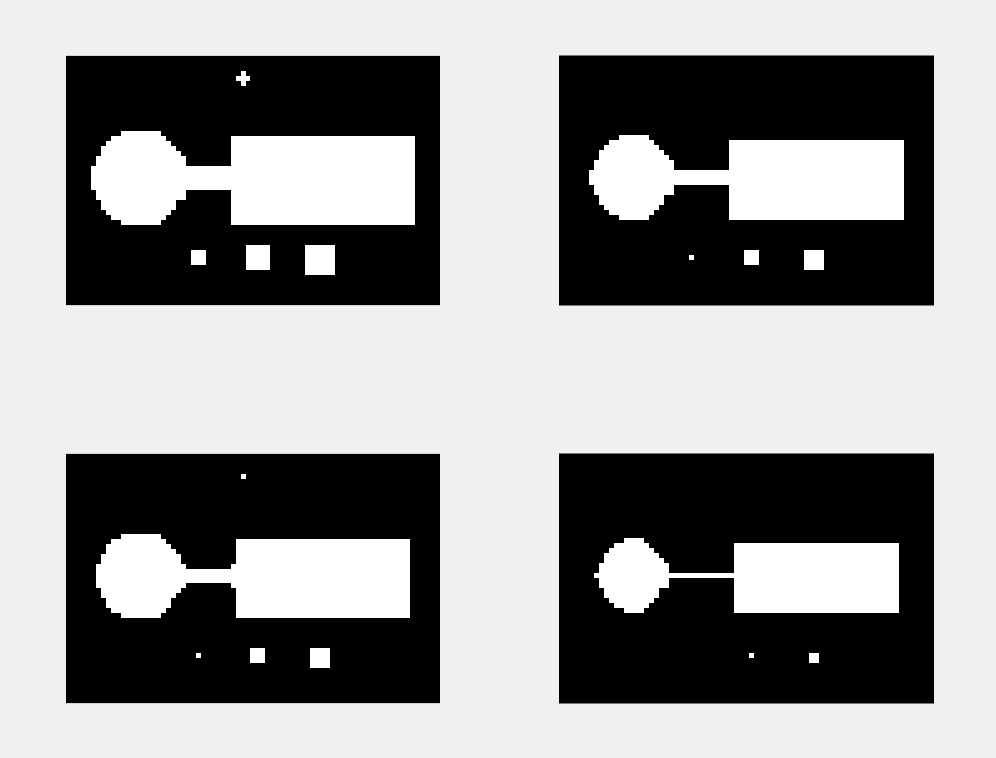#### 膨胀及其实现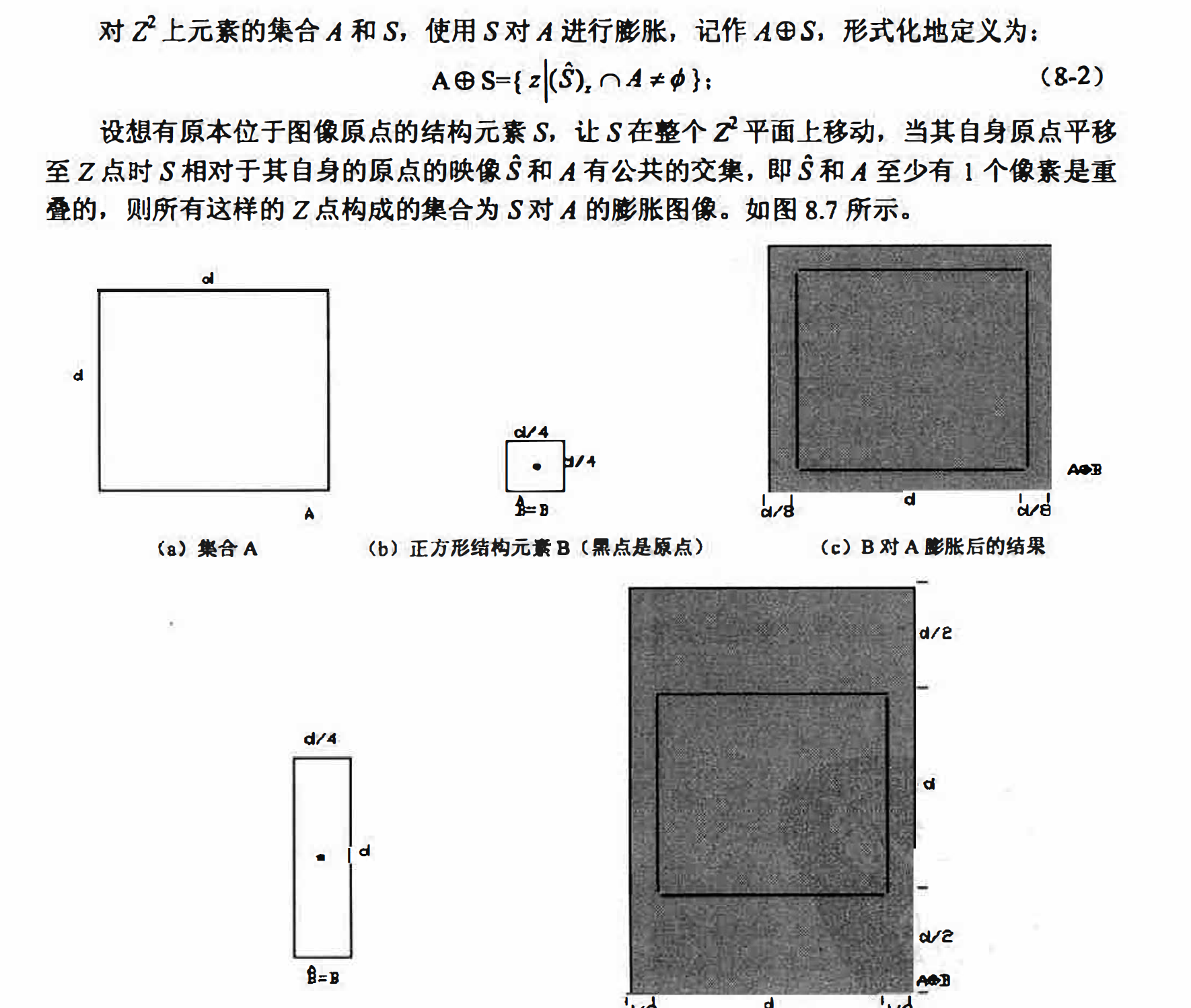matlab实现
imdilate函数用于完成图像膨胀， 其常用调用形式如下：
I2 = imdilate(I,SE);
I为原始图像， 可以是二位或灰度图像（对应于灰度膨胀）．
SE是由strel函数返回的自定义或预设的结构元素对象

I = imread('starcraft.bmp');
Ie1 = imerode(I,[1 1 1;1 1 1;1 1 1]);
Ie2 = imerode(Ie1,[0 1 0;1 1 1;0 1 0]);
Id1 = imdilate(Ie2,[1 1 1;1 1 1;1 1 1]);
Id2 = imdilate(Id1,[0 1 0;1 1 1;0 1 0]);

figure;
subplot(2,2,1);
imshow(Ie1);
subplot(2,2,2);
imshow(Ie2);
subplot(2,2,3);
imshow(Id1);
subplot(2,2,4);
imshow(Id2);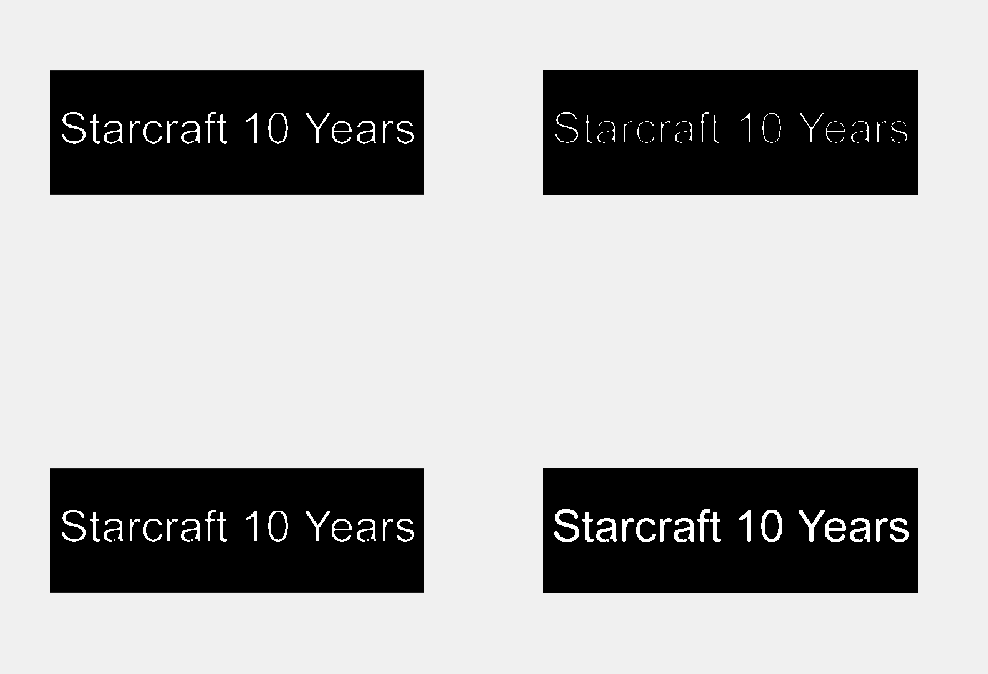#### 开运算及其实现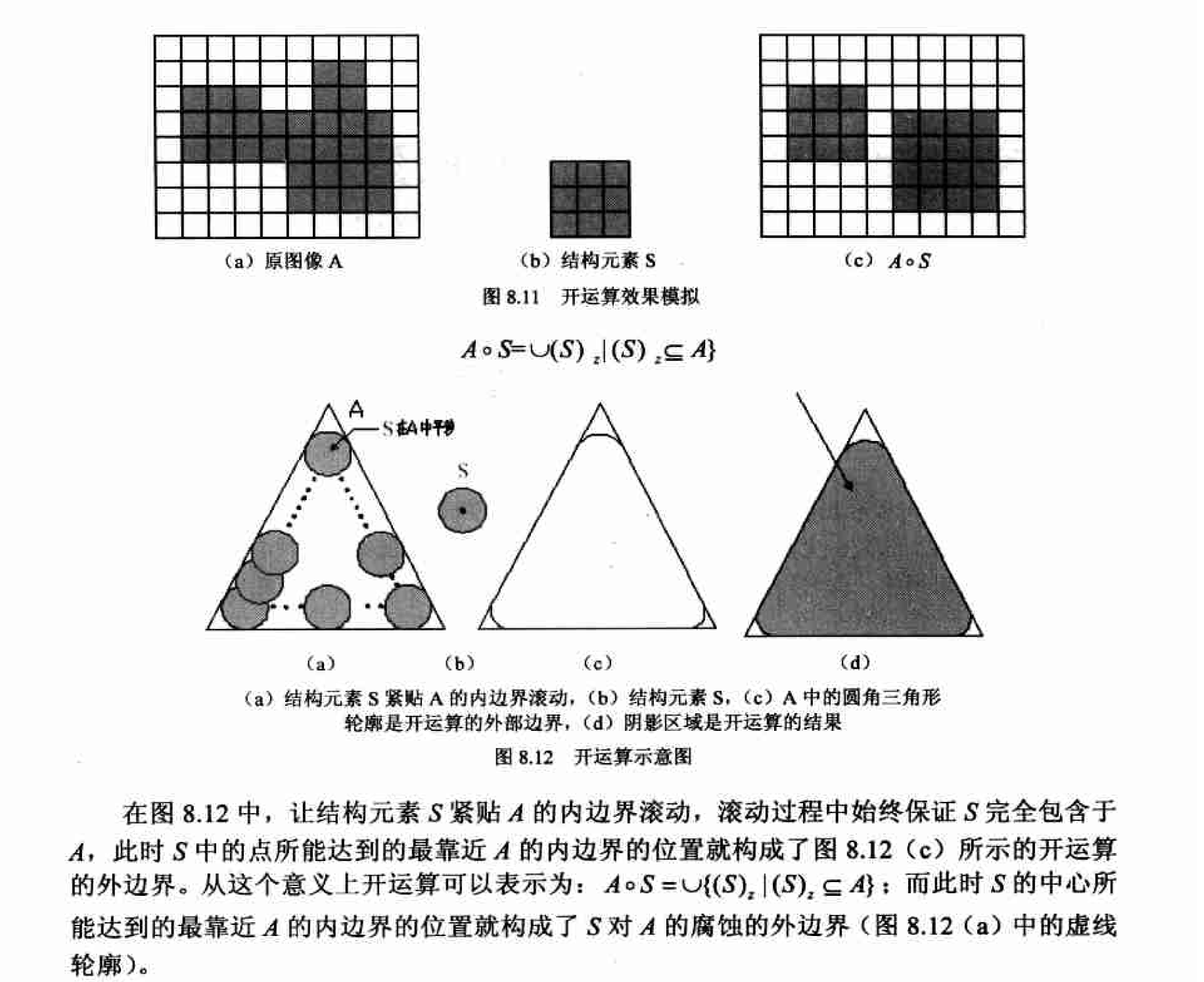matlab实现

I2 = imopen(I,SE);

I = imread('erode_dilate.bmp');
Io = imopen(I,ones(6,6));
figure;
subplot(1,2,1);
imshow(I);
subplot(1,2,2);
imshow(Io);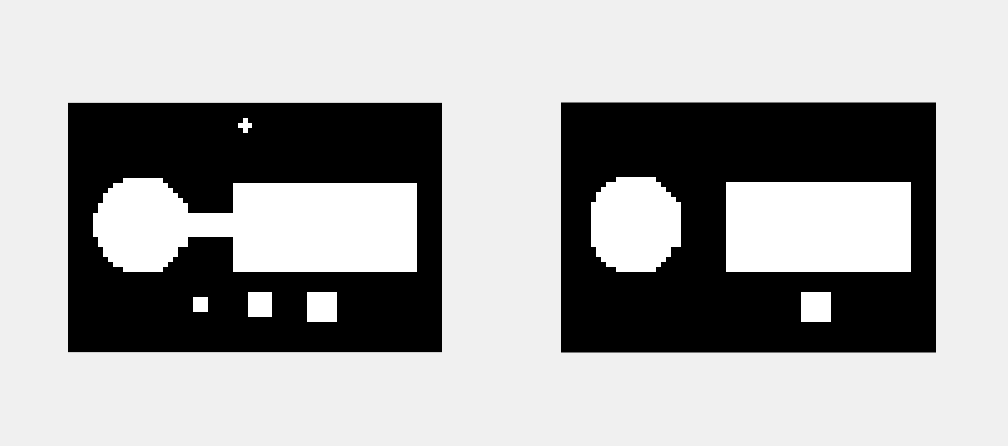从图8.13中可以看到同腐蚀相比，开运算在过滤噪声的同时并没有对物体的形状、轮廓造成明显的影响，这是一大优势。但当我们只关心物体的位置或者个数时，物体形状的改变不会给我们带来困扰，此时用腐蚀滤波具有处理速度上的优势〈同开运算相比节省了一次膨胀运算〉。

#### 闭运算及其实现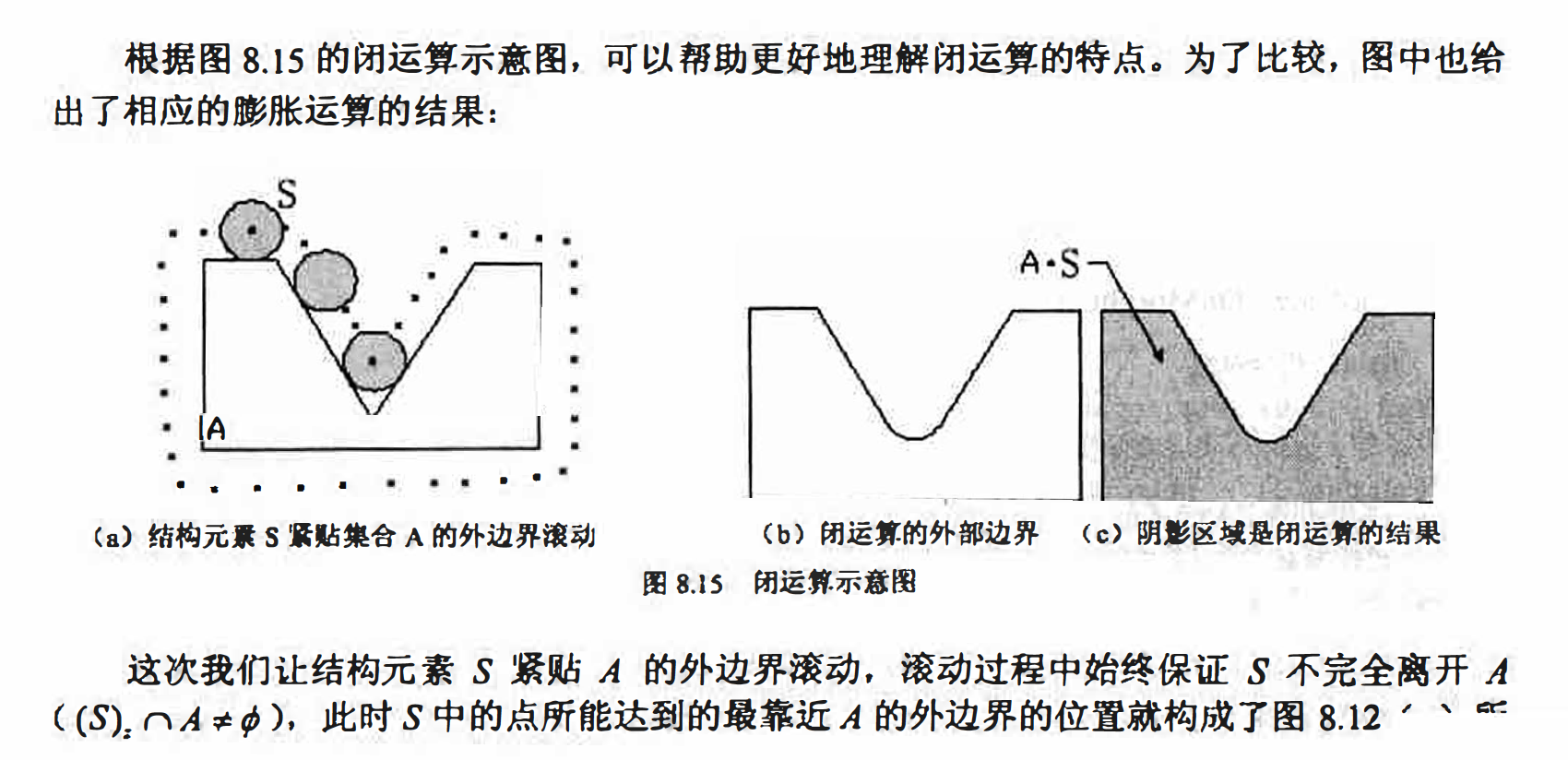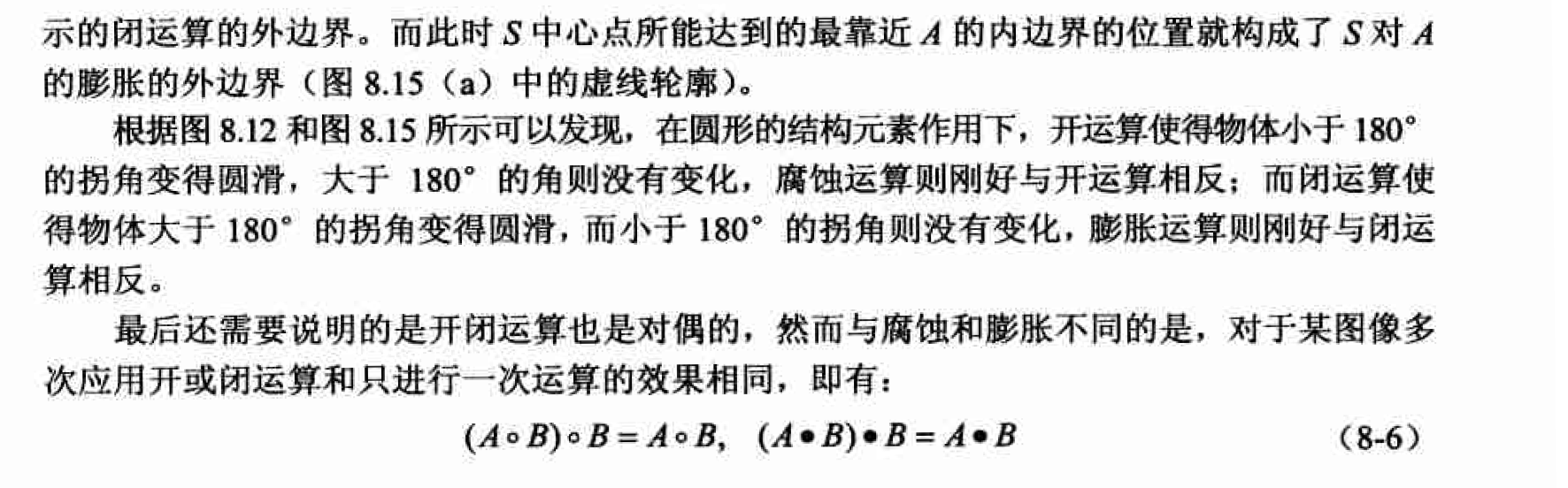Matlab实现

### 二值图像中的形态学应用

#### 击中与击不中交换及其实现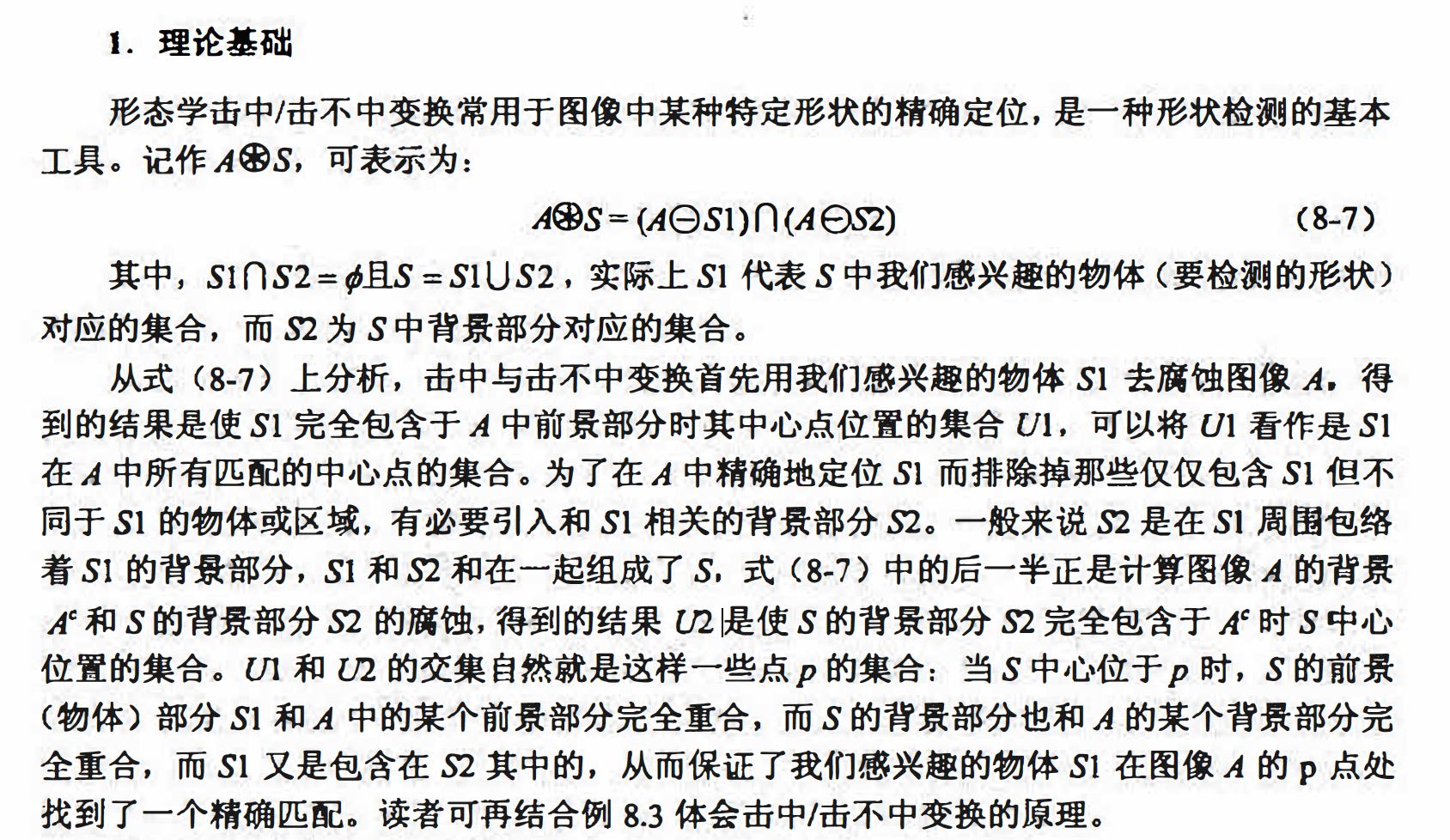I = zeros(120,180);
I(11:80,16:75)=1;
I(56:105,86:135)=1;
I(26:55,141:170)=1;

se = zeros(58,58);
se(5:54,5:54)=1;

Ie1 = imerode(I,se);
Ic = 1 - I;
S2 = 1 - se;
Ie2 = imerode(Ic,S2);
Ihm = Ie1&Ie2;

figure;
subplot(2,2,1);
imshow(I);
subplot(2,2,2);
imshow(Ie1);
subplot(2,2,3);
imshow(Ie2);
subplot(2,2,4);
imshow(Ihm);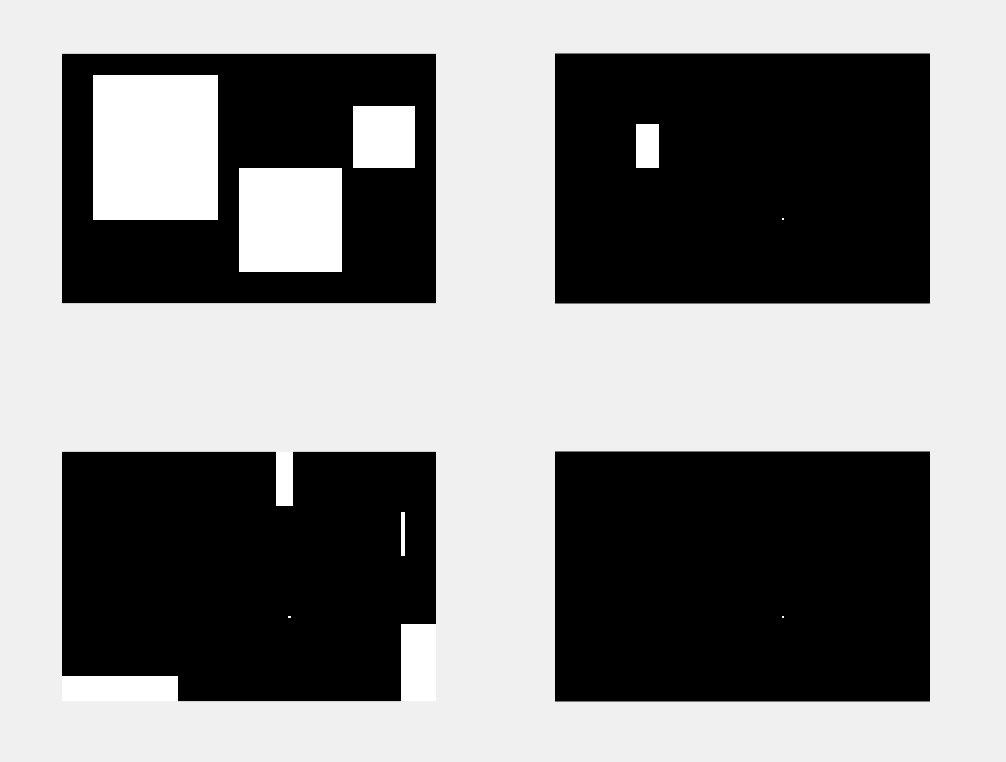#### 边界提取与跟踪及其实现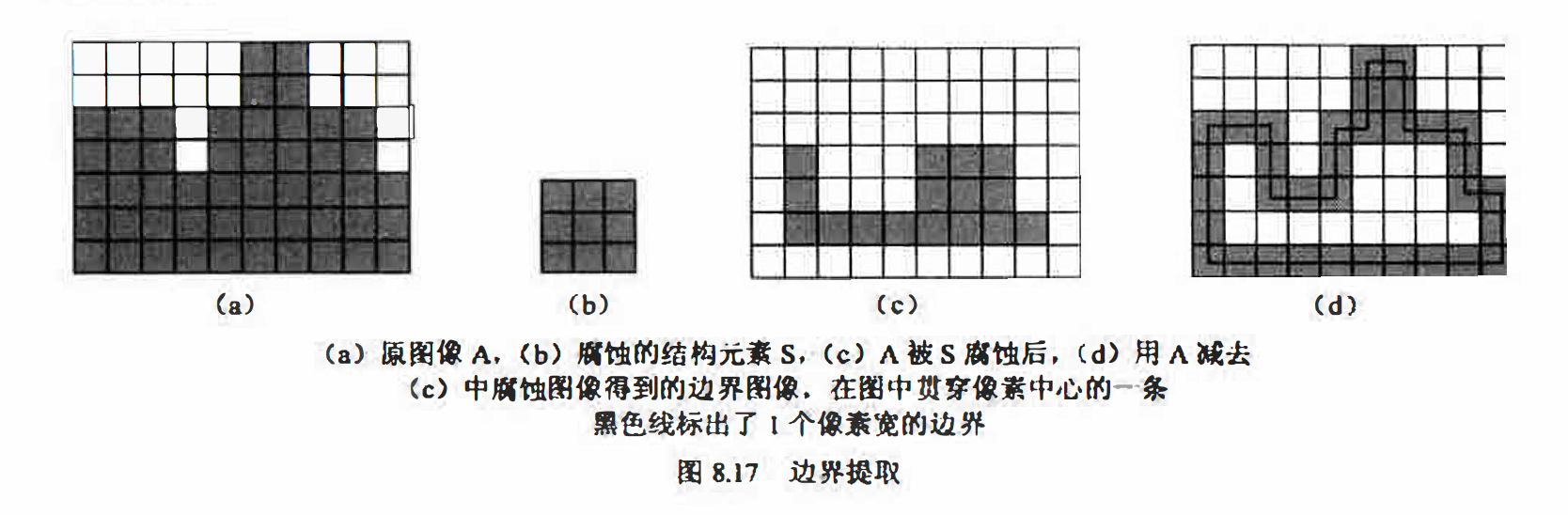I = imread('head_portrait.bmp');
se = strel('square',3);
Ie = imerode(I,se);
Iout = I - Ie;
figure;
subplot(1,2,1);
imshow(I);
subplot(1,2,2);
imshow(Iout);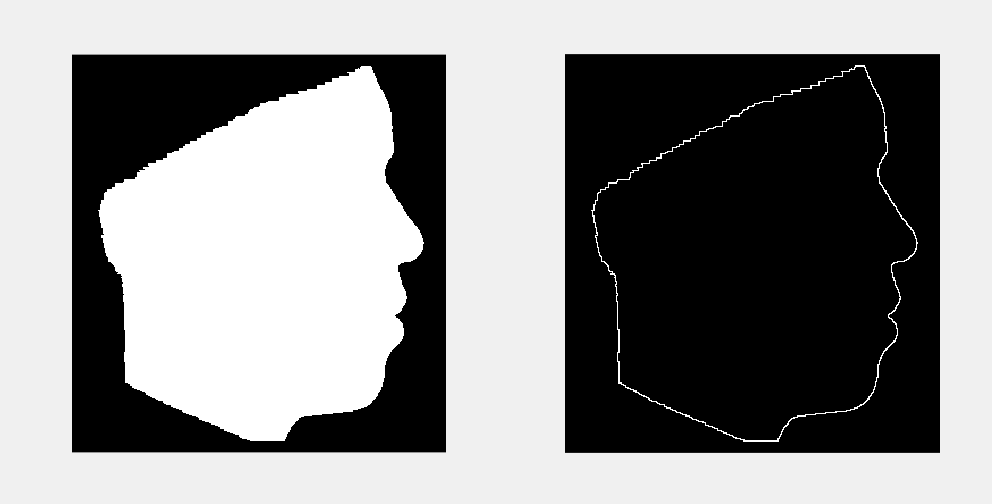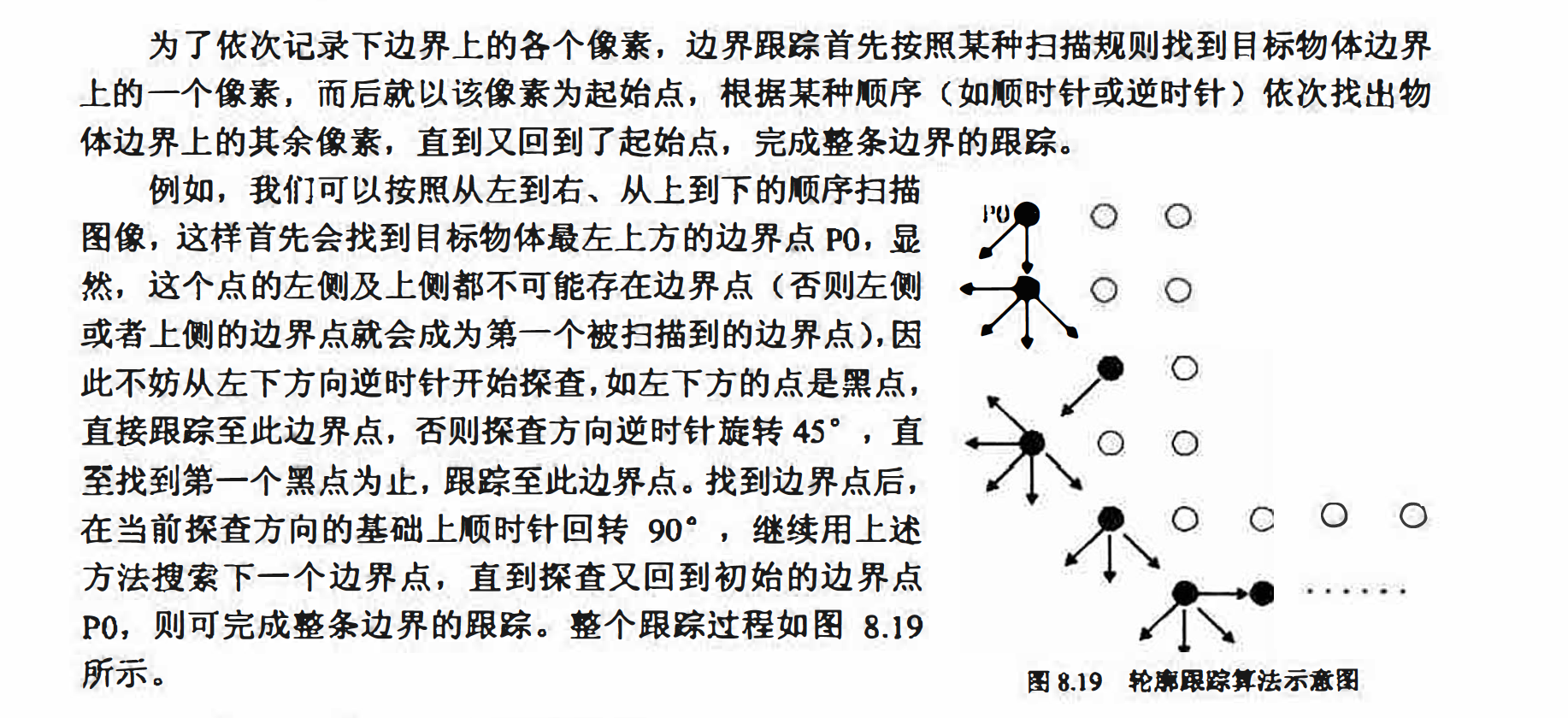#### 区域填充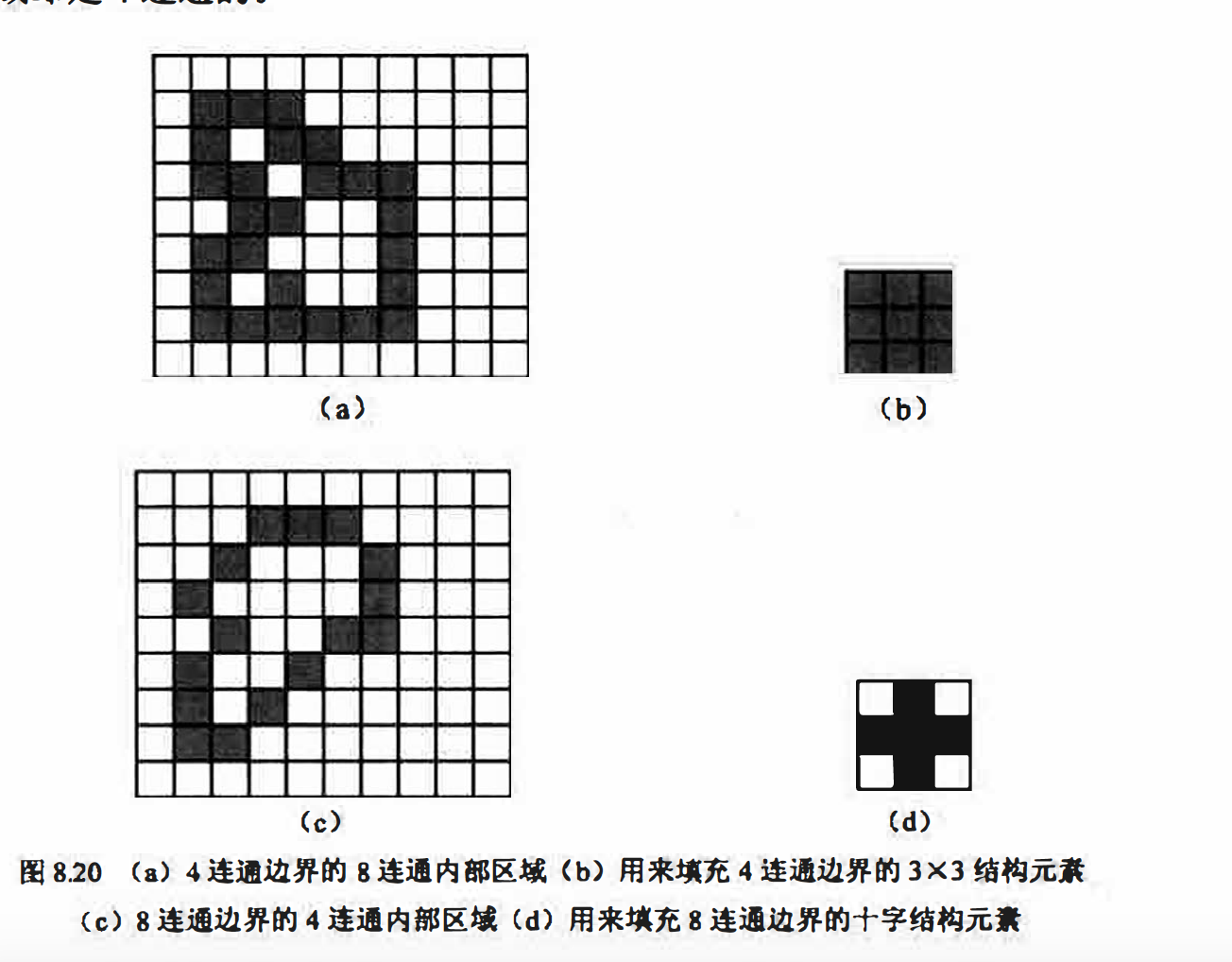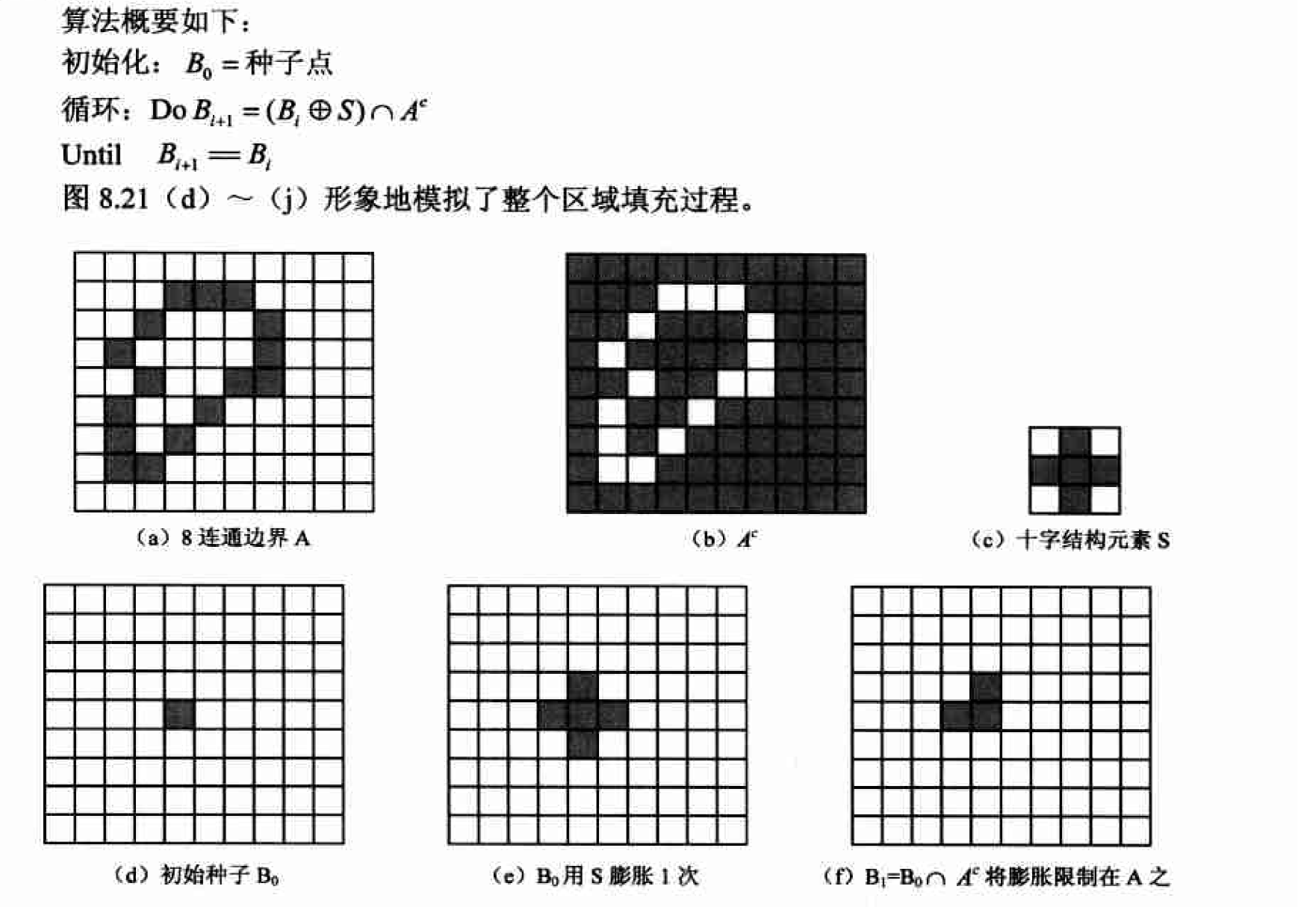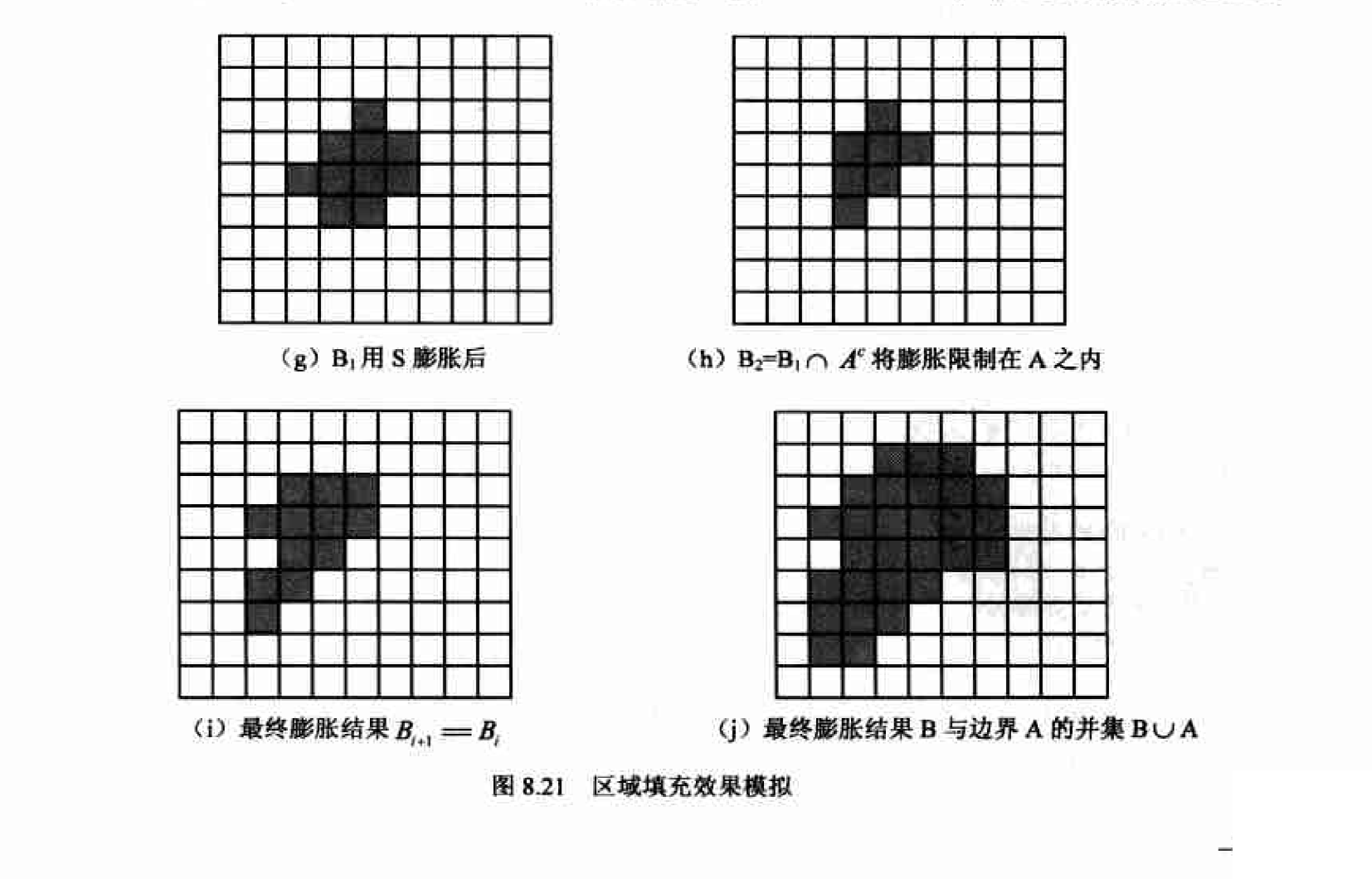#### 连通分量提取及其实现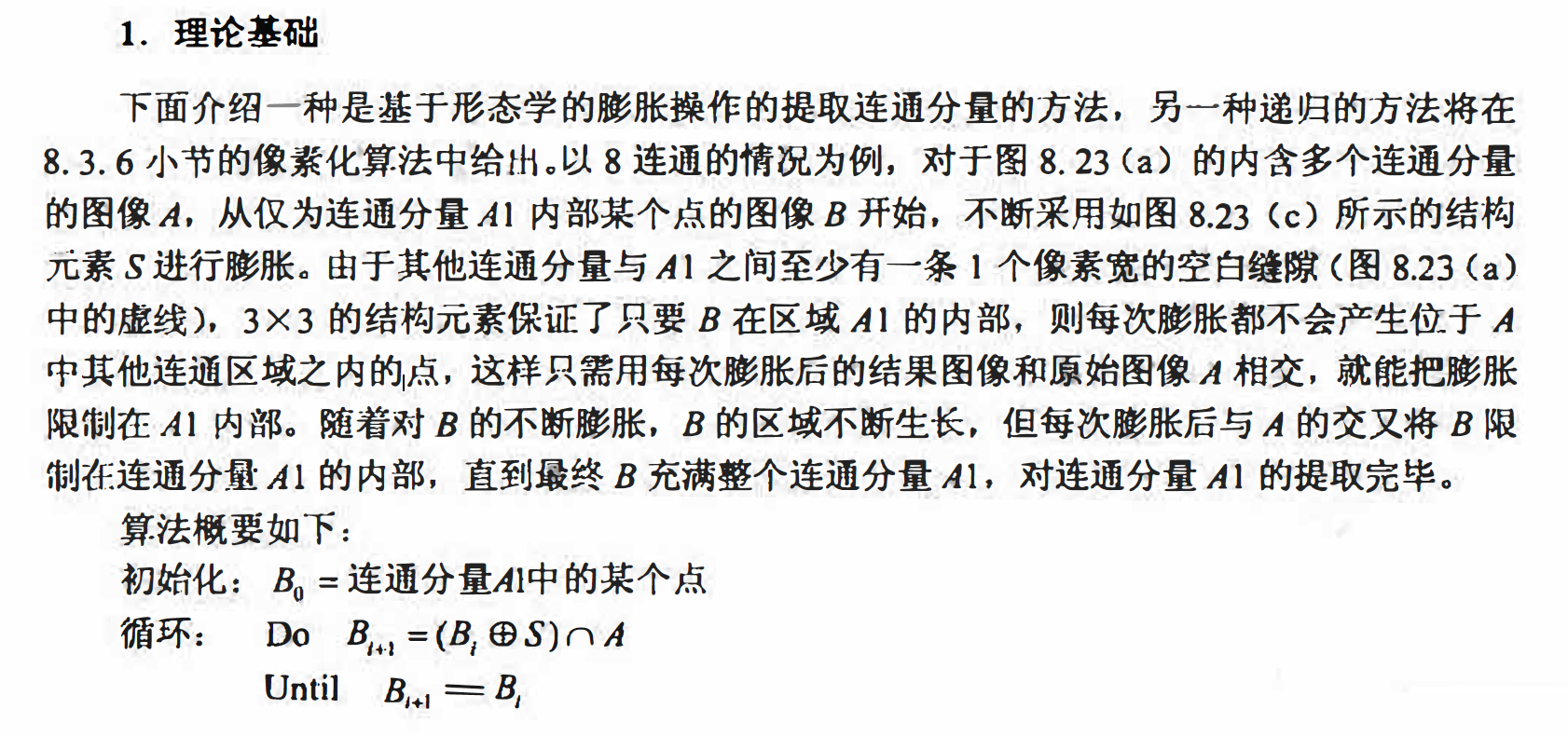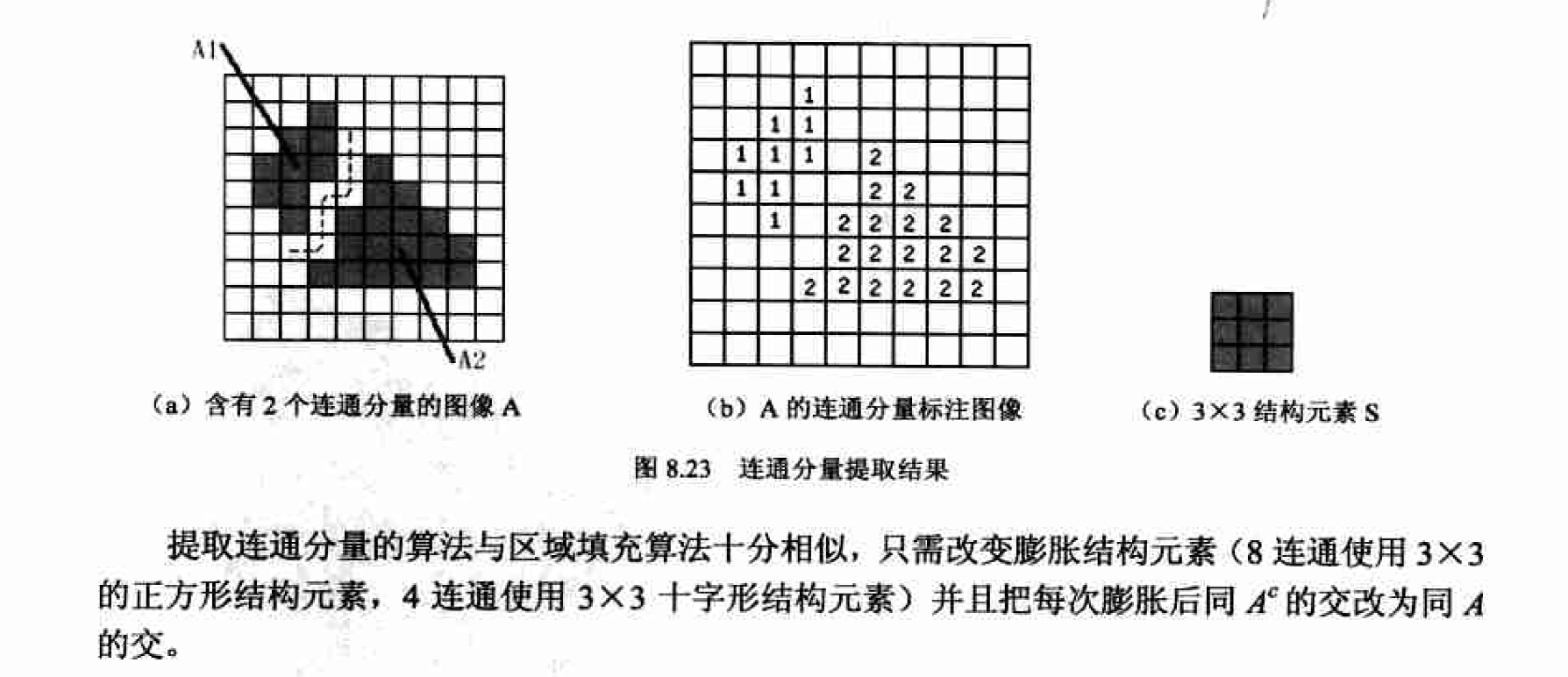matlab实现

[L num]= bwlabel(Ibw,conn);
Ibw为一幅输入二位图像．
conn为可选参数， 指明要提取的连通分量是4连边还是8连通， 默认值为8.
L为类似于图8.23 ( b）的标注图像．
num为二维图像Ibw中连通分量的个数．

(1）对输入图像进行二位化处理．
(2）标注二值图像中的连通分量．
(3）找出最大的连通分量．
(4）计算最大连通分量的中心．

% locateMouth.m

Id = im2double(I);
figure, imshow(Id) % 得到8.24(a)
Ibw = im2bw(Id, 0.38); % 以0.38为阈值二值化
Ibw = 1 - Ibw; %为在Matlab中进行处理，将图像反色
figure, imshow(Ibw) % 得到8.24(b)
hold on
[L, num] = bwlabel(Ibw, 8); % 标注连通分量
disp(['图中共有' num2str(num) '个连通分量'])

% 找出最大的连通分量（嘴）
max = 0; % 当前最大连通分量的大小
indMax = 0; % 当前最大连通分量的索引
for k = 1:num
[y x] = find(L == k); % 找出编号为k的连通区的行索引集合y和列索引集合x

nSize = length(y); %计算该连通区中的像素数目
if(nSize > max)
max = nSize;
indMax = k;
end
end

if indMax == 0
disp('没有找到连通分量')
return
end

% 计算并显示最大连通分量（嘴）的中心
[y x] = find(L == indMax);
yMean = mean(y);
xMean = mean(x);
plot(xMean, yMean, 'Marker', 'o', 'MarkerSize', 14, 'MarkerEdgeColor', 'w', 'MarkerFaceColor', 'w');
plot(xMean, yMean, 'Marker', '*', 'MarkerSize', 12, 'MarkerEdgeColor', 'k'); % 得到8.24(c)


I = imread('bw_bacteria.bmp');
[L,num]=bwlabel(I,8);
Idil = imdilate(I,ones(3,3));
[L,num] = bwlabel(Idil,8);

figure;
subplot(1,2,1);
imshow(I);
subplot(1,2,2);
imshow(Idil);